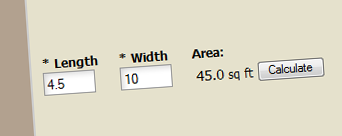# New Calculations Feature

Have you ever needed your form to calculate a value based on a user’s input? With our new Calculations feature, your form can now do this.

For example, if you have a text field for “length” and a text field for “width”, you can set up an equation that multiplies these fields together to calculate a user’s square footage value.Calculations can handle any combination of mathematical operations: addition, subtraction, multiplication, and division. You can also use Calculations to customize Order Form prices and Scoring points.